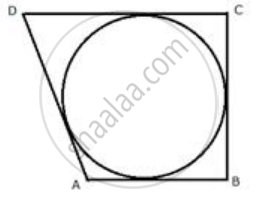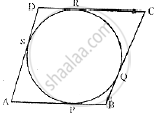Share

# If the Sides of a Parallelogram Touch a Circle (Refer Figure of Q. 7), Prove that the Parallelogram is a Rhombus - Mathematics

Course

#### Question

If the sides of a parallelogram touch a circle in following figure , Prove that the parallelogram is a rhombus.#### SolutionFrom A, AP and AS are tangents to the circle.
Therefore, AP = AS.......(i)
Similarly, we can prove that:
BP = BQ .........(ii)
CR = CQ .........(iii)
DR = DS .........(iv)
AP + BP + CR + DR = AS + DS + BQ + CQ
AB + CD = AD + BC
Hence, AB + CD = AD + BC
But AB = CD and BC = AD.......(v) Opposite sides of a ||gm
Therefore, AB + AB = BC + BC
2AB = 2 BC
AB = BC ........(vi)

From (v) and (vi)
AB = BC = CD = DA
Hence, ABCD is a rhombus

Is there an error in this question or solution?